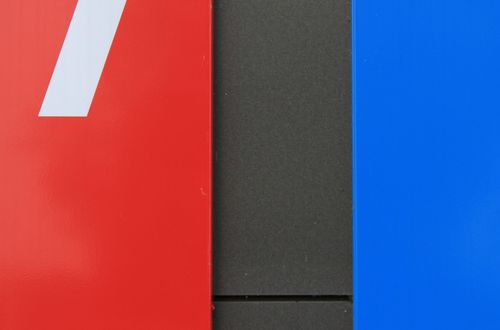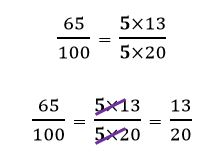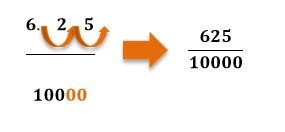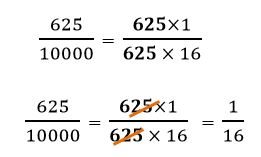# How to turn percentages into fractions

Want to find out how to turn percentages into fractions? We've provided a step-by-step guide of how to turn percentages into fractions to teach you everything you need to know.## Step 1: Express the percentage as a fraction

Express the percentage as a fraction by dividing it with 100.

## Step 2: Write the numerator as a whole number

We then to write the numerator as a whole number by moving the decimal place to the right. Take note of the number of times you moved the decimal places. Add the same number of zeroes on the denominator.

## Step 3: Simplify the fractions

Find common factors shared by the numerator and denominator to simplify the fraction to its lowest terms.

## How to turn percentages into fractions

Q1) What would 65% be in fraction form?

Express 65% as a fraction first: 65/100

Simplify the fraction by finding common factors we cancel out between numerators and denominators.That’s why 65%= 13/20

Q2) What is 6.25% in fraction form?

Divide the percentage by 100 and express in fraction as (6.25)/100 .

Move two decimal places to the left of 6.25 so we end up a whole number as a numerator. Then add two zeroes after 100.Simplify the fraction by cancelling out common factors.Hence, we have 6.25%=1/16 in fraction form.

#### Join today

The fastest way to practice

Unlock our complete testing platform and improve faster that ever.